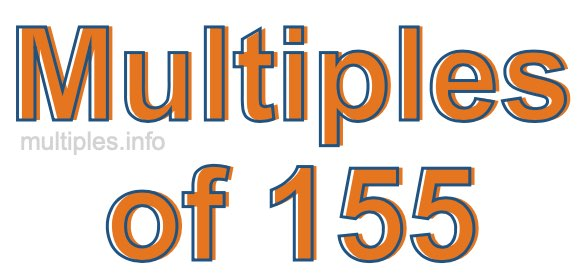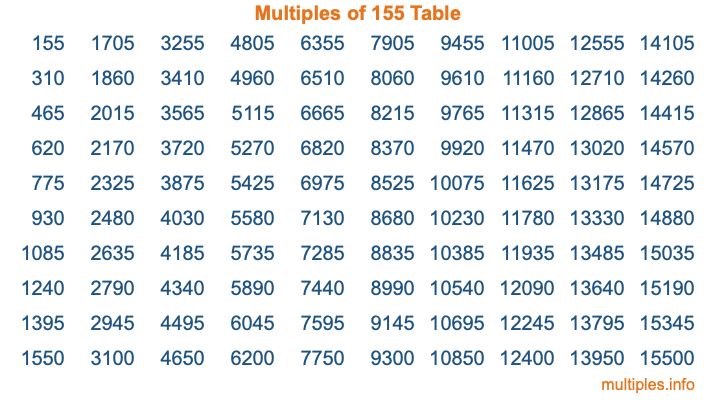Multiples of 155Welcome to the Multiples of 155 page. Here we will first teach you everything you will ever need to know about the multiples of 155, and then give you a study guide summary of everything we taught you to make sure you remember it all. Use this page to look up facts and learn information about the multiples of 155. This page will make you a multiples of one hundred fifty-five expert!

Definition of Multiples of 155
Multiples of 155 are all the numbers that when divided by 155 equal an integer. Each of the multiples of 155 are called a multiple. A multiple of 155 is created by multiplying 155 by an integer.

Therefore, to create a list of multiples of 155, you start with 1 multiplied by 155, then 2 multiplied by 155, then 3 multiplied by 155, and so on for as long as you want. Thus, the list of the first five multiples of 155 is 155, 310, 465, 620, and 775. To see a larger list of multiples of 155, see the printable image of Multiples of 155 further down on this page. We also have a category where you can choose any nth multiple of 155.

Multiples of 155 Checker
The Multiples of 155 Checker below checks to see if any number of your choice is a multiple of 155. In other words, it checks to see if there is any number (integer) that when multiplied by 155 will equal your number. To do that, we divide your number by 155. If the the quotient is an integer, then your number is a multiple of 155.

Is  a multiple of 155?

Least Common Multiple of 155 and ...
A Least Common Multiple (LCM) is the lowest multiple that two or more numbers have in common. This is also called the smallest common multiple or lowest common multiple and is useful to know when you are adding our subtracting fractions. Enter one or more numbers below (155 is already entered) to find the LCM.

Check out our LCM Calculator if you need more details about the Least Common Multiple or if you need the LCM for different numbers for adding and subtraction fractions.

nth Multiple of 155
As we stated above, 155 is the first multiple of 155, 310 is the second multiple of 155, 465 is the third multiple of 155, and so on. Enter a number below to find the nth multiple of 155.

th multiple of 155

Multiples of 155 vs Factors of 155
155 is a multiple of 155 and a factor of 155, but that is where the similarities end. All postive multiples of 155 are 155 or greater than 155. All positive factors of 155 are 155 or less than 155.

Below is the beginning list of multiples of 155 and the factors of 155 so you can compare:

Multiples of 155: 155, 310, 465, 620, 775, etc.

Factors of 155: 1, 5, 31, 155

As you can see, the multiples of 155 are all the numbers that you can divide by 155 to get a whole number. The factors of 155, on the other hand, are all the whole numbers that you can multiply by another whole number to get 155.

It's also interesting to note that if a number (x) is a factor of 155, then 155 will also be a multiple of that number (x).

Multiples of 155 vs Divisors of 155
The divisors of 155 are all the integers that 155 can be divided by evenly. Below is a list of the divisors of 155.

Divisors of 155: 1, 5, 31, 155

The interesting thing to note here is that if you take any multiple of 155 and divide it by a divisor of 155, you will see that the quotient is an integer.

Multiples of 155 Table
Below is an image of the first 100 multiples of 155 in a table. The table is in chronological order, column by column. The first column has the first ten multiples of 155, the second column has the next ten multiples of 155, and so on.The Multiples of 155 Table is also referred to as the 155 Times Table or Times Table of 155. You are welcome to print out our table for your studies.

Negative Multiples of 155
Although not often discussed or needed in math, it is worth mentioning that you can make a list of negative multiples of 155 by multiplying 155 by -1, then by -2, then by -3, and so on, to get the following list of negative multiples of 155:

-155, -310, -465, -620, -775, etc.

Multiples of 155 Summary
Below is a summary of important Multiples of 155 facts that we have discussed on this page. To retain the knowledge on this page, we recommend that you read through the summary and explain to yourself or a study partner why they hold true.

There are an infinite number of multiples of 155.

A multiple of 155 divided by 155 will equal a whole number.

155 divided by a factor of 155 equals a divisor of 155.

The nth multiple of 155 is n times 155.

The largest factor of 155 is equal to the first positive multiple of 155.

155 is a multiple of every factor of 155.

155 is a multiple of 155.

A multiple of 155 divided by a divisor of 155 equals an integer.

155 divided by a divisor of 155 equals a factor of 155.

Any integer times 155 will equal a multiple of 155.

Multiples of a Number
Here you can get the multiples of another number, all with the same attention to detail as we did for multiples of 155 on this page.

Multiples of
Multiples of 156
Did you find our page about multiples of one hundred fifty-five educational? Do you want more knowledge? Check out the multiples of the next number on our list!